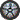### Prof. Paulo Franca - Spring 2000

Introduction

Syllabus

Guidelines for labs

Lab sections

Take quiz & check grades

## Assimptotic Notation

(Chapter 2)

Functions to denote worst-case running-time function T(n), as n (integer) grows to large values.Even though those functions have integer domains, it is common to represent them using a continuous real domain.

#### Teta notation:

Find two functions that will "sandwich" T(n) assimptotically

#### O notation

Find a function that will assimptotically approach the upper bound of T(n)

#### Omega notation

Find a function that will assimptotically approach the lower bound of T(n)

#### o notation and w notation

Similar to O and omega, but not assimptoticlaly tight